# Test: Enginering Mathematics - 1

## 15 Questions MCQ Test GATE ECE (Electronics) 2023 Mock Test Series | Test: Enginering Mathematics - 1

Description
Attempt Test: Enginering Mathematics - 1 | 15 questions in 45 minutes | Mock test for Electronics and Communication Engineering (ECE) preparation | Free important questions MCQ to study GATE ECE (Electronics) 2023 Mock Test Series for Electronics and Communication Engineering (ECE) Exam | Download free PDF with solutions
QUESTION: 1

Solution:
QUESTION: 2

Solution:
QUESTION: 3

### The order of differential equation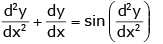Solution:
QUESTION: 4

The order of differential equation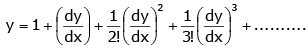Solution:
QUESTION: 5

Find the rank of matrix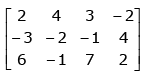Solution:
QUESTION: 6

The rank of Diag matrix  [− 1 0 1 0 0 4] is ________

Solution:
QUESTION: 7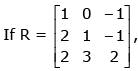the third row of R-1 is

Solution:
QUESTION: 8

For what value of k will the matrix given below become singular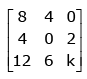is ___________

Solution:

Explanation:- If the matrix is singular, its determinant has to be zero.

[8(0-12) -4(4k-24) +0(24-0)] = 0

-96 -16k +96 = 0

-16k = 0

k = 0

QUESTION: 9

The Eigen values of the matrix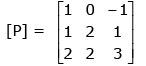Solution:
QUESTION: 10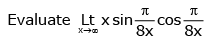Solution:
QUESTION: 11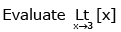Solution:
QUESTION: 12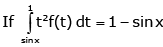then find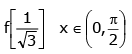Solution:
QUESTION: 13

Given system of linear equations

3x + 2y + z = 0, x + 4y + z = 0, 2x + y + 4z = 0, has

Solution:
QUESTION: 14

Suppose that the probability of event A is 0.2 and the probability of event B is 0.4. Also, suppose that the two events are independent. Then P(A|B) is:

Solution:
QUESTION: 15

The Newton-Raphson method formula for finding the square root of a real number R from the equation x2 − R = 0 is

Solution:Use Code STAYHOME200 and get INR 200 additional OFF Use Coupon Code